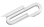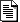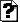# Jim'sTutorials

Spring 2018

## Chris says

Attached is my implementation (and explanation, partly for my own sake) of RK4. I ran out of time to do anything particularly interesting with it, but it works.

## Jim says

Looks good.

If you want to try RK4 on something meaty ... perhaps as a lead in to another topic, check out https://en.wikipedia.org/wiki/Double_pendulum .

Or trajectories in the potential that I did my PhD thesis on :

$$V(x,y) = \left( y - \frac{x^2}{2} \right)^2 + \frac{\mu \, x^2}{2}$$

where $$\mu = 0.1$$.

See

Tangentially related to that : Pollack and fractal dimensions

Chaos : An Introduction to Dynamical Systems - Alligood, Sauer, & Yorke

## attachmentslast modified sizerunge_kutta.html Wed Nov 29 2023 05:45 am 300Krunge_kutta.ipynb Wed Nov 29 2023 05:45 am 42K